### 9-2 Multiword Division

As in the case of multiword multiplication, multiword division may be done by, basically, the traditional grade-school method. The details, however, are surprisingly complicated. Figure 9-1 is Knuth's Algorithm D [Knu2 sec. 4.3.1], coded in C. The underlying form of division it uses is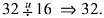(Actually, the quotient of these underlying division operations is at most 17 bits long.)

##### Figure 9-1 Multiword integer division, unsigned , .
`int divmnu(unsigned short q[], unsigned short r[], `
`牋牋燾onst unsigned short u[], const unsigned short v[], `
`牋牋爄nt m, int n) {`
` `
`牋 const unsigned b = 65536; // Number base (16 bits). `
`牋爑nsigned short *un, *vn;?// Normalized form of u, v. `
`牋爑nsigned qhat;牋牋牋牋牋?// Estimated quotient digit. `
`牋爑nsigned rhat;牋牋牋牋牋?// A remainder. `
`牋爑nsigned p;牋牋牋牋牋牋牋 // Product of two digits. `
`牋爄nt s, i, j, t, k; `
` `
`牋 if (m < n || n <= 0 || v[n-1] == 0) `
`牋牋牋return 1;牋牋牋牋牋牋?// Return if invalid param. `
` `
`牋 if (n == 1) {牋牋牋牋牋牋牋牋牋牋牋 ?/ Take care of `
`牋牋牋k = 0;牋牋牋牋牋牋牋牋牋牋牋牋牋?// the case of a `
`牋牋牋for (j = m - 1; j >= 0; j--) {牋?// single-digit `
```牋牋牋牋?span
lang=EN-GB>q[j] = (k*b + u[j])/v;牋牋?// divisor here. ```
`牋牋牋牋爇 = (k*b + u[j]) - q[j]*v; `
`牋牋牋} `
`牋牋牋if (r != NULL) r = k; `
`牋牋牋return 0; `
`牋爙 `
` `
`牋 // Normalize by shifting v left just enough so that `
`牋?/ its high-order bit is on, and shift u left the `
`牋?/ same amount. We may have to append a high-order `
`牋?/ digit on the dividend; we do that unconditionally. `
` `
`牋 s = nlz(v[n-1]) - 16;牋牋牋?// 0 <= s <= 16. `
`牋爒n = (unsigned short *)alloca(2*n); `
`牋?/span>for (i = n - 1; i > 0; i--) `
`牋牋牋vn[i] = (v[i] << s) | (v[i-1] >> 16-s); `
`牋爒n = v << s; `
` `
`牋 un = (unsigned short *)alloca(2*(m + 1)); `
`牋爑n[m] = u[m-1] >> 16-s; `
`牋爁or (i = m - 1; i > 0; i--) `
`牋牋牋un[i] = (u[i] << s) | (u[i-1] >> 16-s); `
`牋爑n = u << s; `
`牋爁or (j = m - n; j >= 0; j--) {牋牋牋 // Main loop. `
`牋牋牋// Compute estimate qhat of q[j]. `
`牋牋牋qhat = (un[j+n]*b + un[j+n-1])/vn[n-1]; `
`牋牋牋rhat = (un[j+n]*b + un[j+n-1]) - qhat*vn[n-1]; `
`again: `
`牋牋牋if (qhat >= b || qhat*vn[n-2] > b*rhat + un[j+n-2]) `
`牋牋牋{ qhat = qhat - 1; `
`牋牋牋牋rhat = rhat + vn[n-1]; `
`牋牋牋牋if (rhat < b) goto again; `
`牋牋牋} `
` `
`牋牋?// Multiply and subtract. `
`牋牋牋k = 0; `
`牋牋牋for (i = 0; i < n; i++) {`
`牋牋牋牋 p = qhat*vn[i]; `
`牋牋牋牋爐 = un[i+j] - k - (p & 0xFFFF); `
`牋牋牋牋爑n[i+j] = t; `
`牋牋牋牋爇 = (p >> 16) - (t >> 16); `
`牋牋牋} `
`牋牋牋t = un[j+n] - k; `
`牋牋牋un[j+n] = t; `
` `
`牋牋?q[j] = qhat;牋牋牋牋牋牋?// Store quotient digit. `
`牋牋牋if (t < 0) {牋牋牋牋牋牋?// If we subtracted too `
`牋牋牋牋爍[j] = q[j] - 1;牋牋牋 // much, add back. `
`牋牋牋牋爇 = 0; `
`牋牋牋牋爁or (i = 0; i < n; i++) {`
`牋牋牋牋牋?t = un[i+j] + vn[i] + k; `
`牋牋牋牋牋牋un[i+j] = t; `
`牋牋牋牋牋牋k = t >> 16; `
`牋牋牋牋爙 `
`牋牋牋牋爑n[j+n] = un[j+n] + k; `
`牋牋牋} `
`牋爙 // End j. `
`牋?/ If the caller wants the remainder, unnormalize `
`牋?/ it and pass it back. `
`牋爄f (r != NULL) {`
`牋牋?for (i = 0; i < n; i++) `
`牋牋牋牋爎[i] = (un[i] >> s) | (un[i+1] << 16-s); `
`牋爙 `
`牋爎eturn 0; `
`} `

The algorithm processes its inputs and outputs a halfword at a time. Of course, we would prefer to process a fullword at a time, but it seems that such an algorithm would require an instruction that does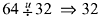division. We assume here that either the machine does not have that instruction or it is hard to access from our high-level language. Although we generally assume the machine has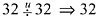division, for this problem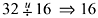suffices.

Thus, for this implementation of Knuth's algorithm, the base b is 65536. See [Knu2] for most of the explanation of this algorithm.

The dividend u and the divisor v are in "little-endian" order梩hat is, u  and v  are the least significant digits. (The code works correctly on both big-and little-endian machines.) Parameters m and n are the number of halfwords in u and v, respectively (Knuth defines m to be the length of the quotient). The caller supplies space for the quotient q and, optionally, for the remainder r. The space for the quotient must be at least m - n + 1 halfwords, and for the remainder, n halfwords. Alternatively, a value of NULL can be given for the address of the remainder to signify that the remainder is not wanted.

The algorithm requires that the most significant digit of the divisor, v[n-1], be nonzero. This simplifies the normalization steps and helps to ensure that the caller has allocated sufficient space for the quotient. The code checks that v[n-1] is nonzero, and also the requirements that n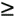1 and mn. If any of these conditions are violated, it returns with an error code (return value 1).

After these checks, the code performs the division for the simple case in which the divisor is of length 1. This case is not singled out for speed; the rest of the algorithm requires that the divisor be of length 2 or more.

If the divisor is of length 2 or more, the algorithm normalizes the divisor by shifting it left just enough so that its high-order bit is 1. The dividend is shifted left the same amount, so the quotient is not changed by these shifts. As explained by Knuth, these steps are necessary to make it easy to guess each quotient digit with good accuracy. The number of leading zeros function, nlz(x), is used to determine the shift amount.

In the normalization steps, new space is allocated for the normalized dividend and divisor. This is done because it is generally undesirable, from the caller's point of view, to alter these input arguments, and because it may be impossible to alter them梩hey may be constants in read-only memory. Furthermore, the dividend may need an additional high-order digit. C's "alloca" function is ideal for allocating this space. It is usually implemented very efficiently, requiring only two or three in-line instructions to allocate the space and no instructions at all to free it. The space is allocated on the program's stack, in such a way that it is freed automatically upon subroutine return.

In the main loop, the quotient digits are cranked out one per loop iteration, and the dividend is reduced until it becomes the remainder. The estimate qhat of each quotient digit, after being refined by the steps in the loop labelled again, is always either exact or too high by 1.

The next steps multiply qhat by the divisor and subtract the product from the current remainder, as in the grade school method. If the remainder is negative, it is necessary to decrease the quotient digit by 1 and either re-multiply and subtract or, more simply, adjust the remainder by adding the divisor to it. This need be done at most once, because the quotient digit was either exact or 1 too high.

Lastly, the remainder is given back to the caller if the address of where to put it is non-null. The remainder must be shifted right by the normalization shift amount s.

The "add back" steps are executed only rarely. To see this, observe that the first calculation of each estimated quotient digit qhat is done by dividing the most significant two digits of the current remainder by the most significant digit of the divisor. The steps in the "again" loop amount to refining qhat to be the result of dividing the most significant three digits of the current remainder by the most significant two digits of the divisor (proof omitted; convince yourself of this by trying some examples using b = 10). Note that the divisor is greater than or equal to b/2 (because of normalization) and the dividend is less than or equal to b times the divisor (because each remainder is less than the divisor).

How accurate is the quotient estimated by using only three dividend digits and two divisor digits? Because normalization was done, it can be shown to be quite accurate. To see this somewhat intuitively (not a formal proof), consider estimating u/v in this way for base ten arithmetic. It can be shown that the estimate is always high (or exact). Thus, the worst case occurs if truncation of the divisor to two digits decreases the divisor by as much as possible in the sense of relative error, and truncation of the dividend to three digits increases it by as little as possible (which is 0), and if the dividend is as large as possible. This occurs for the case 49900?/5099?, which we estimate by 499/50 = 9.98. The true result is approximately 499/51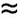9.7843. The difference of 0.1957 reveals that the estimated quotient digit and the true quotient digit, which are the floor functions of these ratios, will differ by at most 1, and this will occur about 20% of the time (assuming the quotient digits are uniformly distributed). This in turn means that the "add back" steps will be executed about 20% of the time.

Carrying out this (non-rigorous) analysis for a general base b yields the result that the estimated and true quotients differ by at most 2/b. For b = 65536, we again obtain the result that the difference between the estimated and true quotient digits is at most 1, and this occurs with probability 2/655360.00003. Thus the "add back" steps are executed for only about 0.003% of the quotient digits.

An example that requires the add back step is, in decimal, 4500/501. A similar example for base 65536 is 0x7FFF 800000000000/0x800000000001.

We will not attempt to estimate the running time of this entire program, but simply note that for large m and n, the execution time is dominated by the multiply/subtract loop. On a good compiler this will compile into about 16 basic RISC instructions, one of which is multiply. The "for j" loop is executed n times, and the multiply/subtract loop m - n + 1 times, giving an execution time for this part of the program of (15 + mul)n(m - n + 1) cycles, where mul is the time to multiply two 16-bit variables. The program also executes m - n + 1 divide instructions and one number of leading zeros instruction.

#### Signed Multiword Division

We do not give an algorithm specifically for signed multiword division, but merely point out that the unsigned algorithm can be adapted for this purpose as follows:

1.       Negate the dividend if it is negative, and similarly for the divisor.

2.       Convert the dividend and divisor to unsigned representation.

3.       Use the unsigned multiword division algorithm.

4.       Convert the quotient and remainder to signed representation.

5.       Negate the quotient if the dividend and divisor had opposite signs.

6.       Negate the remainder if the dividend was negative.

These steps sometimes require adding or deleting a most significant digit. For example, assume for simplicity that the numbers are represented in base 256 (one byte per digit), and that in the signed representation, the high-order bit of the sequence of digits is the sign bit. This is much like ordinary two's-complement representation. Then, a divisor of 255, which has signed representation 0x00FF, must be shortened in step 2 to 0xFF. Similarly, if the quotient from step 3 begins with a 1-bit, it must be provided with a leading 0-byte for correct representation as a signed quantity.